# Free Vibration of Cantilever Beam with Lumped Mass at Free End

2.3 Theoretical natural frequency for cantilever beam

###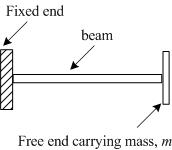Fig. 2.1 (a): A cantilever beam

###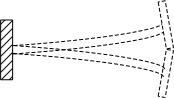Fig. 2.1 (b): The beam under free vibration

A cantilever beam with rectangular cross-section is shown in Fig. 2.1(a). Bending vibration can be generated by giving an initial displacement at the free end of the beam. Fig. 2.1(b) shows a cantilever beam undergoing a free vibration.

When a system is subjected to free vibration and the system is considered as a discrete system in which the beam is considered as mass-less and the whole mass is concentrated at the free end of the beam. The governing equation of motion for such system will be,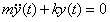(2.1)

Where m is a concentrated mass at the free end of the beam and k is the stiffness of the system. The transverse stiffness of a cantilever beam is given as (using strength of materials deflection formula, Timoshenko and Young, 1961),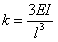(2.2)

Where E is the Young’s modulus of the beam material (it can be obtained by the tensile test of the standard specimen). The fundamental undamped circular natural frequency of the system is given as,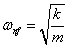(2.3)

Where, m is an equivalent mass placed at the free end of the cantilever beam (of the beam and sensor masses). By substituting equation 2.2 into equation 2.3  we get,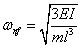(2.4)

The undamped natural frequency is related with the circular natural frequency as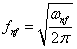(2.5)

The moment of inertia of the beam cross-section and for a circular cross-section it is given as,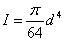(2.6)

Where, d is the diameter of cross section, and for a rectangular cross section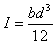(2.7)

Where b and d are the breadth and depth of the beam cross-section as shown in Fig. 2.2. Dimensions of the beam material are given in Table 2.2

###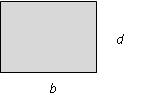Fig. 2.2: A rectangular cross-cross of the beam

In case of the test specimen, the beam mass is distributed over the length. However, by taking one-third of the total mass of beam at the free end (Thompson. 1961), the system can be assumed as discrete system. Hence,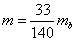(2.8)

Where   m b is the mass of beam and is given as

###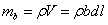Where , ρ is the mass density of the beam material and V is the volume of the beam from the fixed end to the free end.

The equivalent tip mass of a cantilever beam can be obtained as follows. Consider a cantilever beam as shown in Fig. 2.3 (a). Let be m 1 the mass of the beam per unit length, l is the length of the beam, m b =m 1 l is total mass of the beam, and V max is the transverse velocity at the free end of beam and f is the force applied, E is the young’s modulus of the beam and I is the moment of inertia of the beam.

###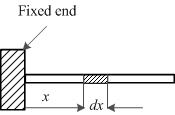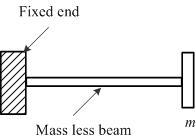Fig. 2.3(a): A cantilever beam with distributed m                Fig. 2.3(b): The cantilever beam with a tip mass

Consider a small element of length dx at a distance x from the free end (Fig. 2.3 (a)). The beam displacement at this point is given by (Timoshenko and Young, 1961),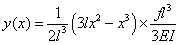(2.9)

Here fl 3 / 3EI is the deflection at free end of the cantilever beam. Now the velocity of the small element at distance x is given by,

###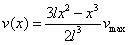Hence, the kinetic energy of the element is given by

###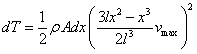and the total kinetic energy of the beam  is

###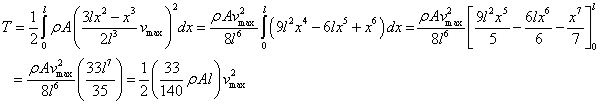(2.10)

where  m 1 l = m b . If we place a mass of 33 / 140m b at the free end of the beam and the beam is assumed to be of negligible mass, then

Total kinetic energy possessed by the beam =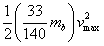(2.11)

Hence two systems are dynamically same. Therefore, the continuous system of cantilever beam can be changed to single degree freedom system as shown in Fig. 2.3(b) by adding the 33  / 140m b of mass at its free end.

Values of the mass density for various beam materials are given in Table 2.1. If any contacting type of transducer is used for the vibration measurement, it should be placed at end of the beam and then the mass of transducer has to be added into the equivalent mass of the beam at the free end of the beam during the natural frequency calculation. If m t is the mass of transducer, then the total mass at the free end of the cantilever beam is given as,